ISEE Middle Level Math : How to find the square root

Example Questions

Example Question #281 : Squares / Square Roots

Solve: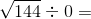undefinedundefined

Explanation:

Division of a non-zero number by 0 is always undefined. Therefore, there is no need to find the square root, because the answer will be undefined regardless of what number is under the square root sign.

Example Question #282 : Squares / Square Roots

Solve: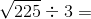Explanation:

First, find the square root: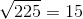Then complete the division: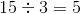Answer:Example Question #283 : Squares / Square Roots

Solve the equation: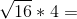Explanation:

First find the square root: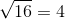Then multiply: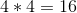Answer:Example Question #284 : Squares / Square Roots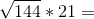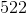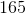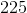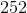Explanation:

First take the square root: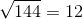Then multiply: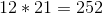Answer:Example Question #285 : Squares / Square Roots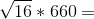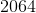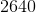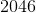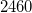Explanation:

First take the square root:Then multiply: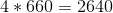Answer:Example Question #286 : Squares / Square Roots

What is the simplified version of this expression: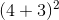Explanation:

The first step in solving this problem is to solve for the value in parentheses: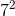This is equivalent to 7 times 7, which is 49. Therefore, 49 is the correct answer.

Example Question #287 : Squares / Square Roots

What is the value of the following expression?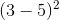Explanation:

The first step in solving this problem is to solve for the value in parentheses: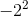Remember, a negative raised to an even power is positive. Therefore, 4 is the correct answer.

Example Question #288 : Squares / Square Roots

Which of the values below is equal to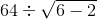?Explanation:can be reduced to: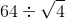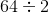Therefore,is the correct answer.

Example Question #289 : Squares / Square Roots

Simplify the following: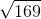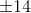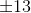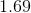Explanation:

To solve, simply identify the number that when squared is equal to 169.

Recall that both negative and positive numbers when squared result in a positive number.

Thus,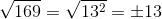Example Question #290 : Squares / Square Roots

What is the side length of a square yard if the area of the yard is?Explanation:

The area of a square is the side length squared.

To find the side length from the area, you must find the square root of the area.

The square root whatever value multiplied by itself gives you your value.multiplied by itself gives youso the side length must be.